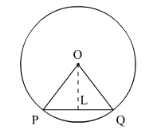# A chord PQ of length 12 cm subtends an angle`
Question:

A chord PQ of length 12 cm subtends an angle of 120° at the centre of a circle. Find the area of the minor segment cut off by the chord PQ.

Solution:

We know that the area of minor segment of angle θ in a circle of radius r is,

$A=\left\{\frac{\pi \theta}{360^{\circ}}-\sin \frac{\theta}{2} \cos \frac{\theta}{2}\right\} r^{2}$

It is given that the chord PQ divides the circle in two segments.We have $\angle P O Q=120^{\circ}$ and $P Q=12 \mathrm{~cm}$. So,

$P L=\frac{P Q}{2} \mathrm{~cm}$

$=\frac{12}{2} \mathrm{~cm}$

$=6 \mathrm{~cm}$

Since $\angle P O Q=120^{\circ}$,

$\angle P O L=\angle Q O L$

$=60^{\circ}$

$\ln \triangle O P Q$, we have

$\sin \theta=\frac{P L}{O A}$

$\sin 60^{\circ}=\frac{6}{O A}$

$\frac{\sqrt{3}}{2}=\frac{6}{O A}$

$O A=\frac{12}{\sqrt{3}}$

Thus the radius of circle is $O A=4 \sqrt{3} \mathrm{~cm}$

Now using the value of radius r and angle θ we will find the area of minor segment

$A=\left\{\frac{120^{\circ} \pi}{360^{\circ}}-\sin \frac{120^{\circ}}{2} \cos \frac{120^{\circ}}{2}\right\}(4 \sqrt{3})^{2}$

$=\left\{\frac{\pi}{3}-\frac{\sqrt{3}}{2} \times \frac{1}{2}\right\} \times 48$

$=4\{4 \pi-3 \sqrt{3}\} \mathrm{cm}^{2}$